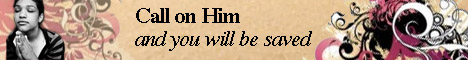Note:  Do not rely on this information. It is very old.

# Levers

Levers are mechanical devices used for transmitting and altering the direction and magnitude of forces. If a bar can turn on a pivot or fulcrum near one end, and a, downward force is applied to the long end, the short end will be raised with a greater force and through a correspondingly smaller distance, as when a crow-bar is used for lifting a heavy body. Call the force applied to one point on the lever P, and the force available at some other point W; then if the fulcrum is between the P and W ends of the lever, W will act in a contrary direction to P, and the magnitude of W will be to the magnitude of P as P's distance from the fulcrum is to W's distance. If W is between the fulcrum and P, or vice versa, the two forces will act in the same direction, and their magnitudes will still be precisely proportional to their distances from the fulcrum. A bell-crank lever is bent at right angles, the fulcrum being at the angle, and a force applied at one end is transmitted at right angles to its original direction. In any lever or system of levers, the force applied at one point, multiplied by the distance through which it acts must - neglecting the effects of friction at the pivot and of bending in the lever - be equal to the force developed at any other point multiplied by the distance through which it acts.